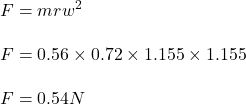## An object with mass m = 0.56 kg is attached to a string of length r = 0.72 m and is rotating with an angular velocity ω = 1.155 rad/s. What

Question

An object with mass m = 0.56 kg is attached to a string of length r = 0.72 m and is rotating with an angular velocity ω = 1.155 rad/s. What is the centripetal force acting in the object?

in progress 0
6 months 2021-07-23T05:24:57+00:00 1 Answers 14 views 0

## Answers ( )

The centripetal force is 0.54 N.

Explanation:

mass, m = 0.56 kg

radius, r = 0.72 m

angular speed, w = 1.155 rad/s

The centripetal force is given by